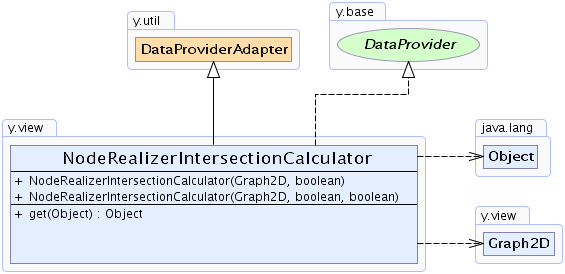Search this API

## y.view Class NodeRealizerIntersectionCalculator

```java.lang.Objecty.util.DataProviderAdaptery.view.NodeRealizerIntersectionCalculator
```
All Implemented Interfaces:
DataProvider

`public class NodeRealizerIntersectionCalculatorextends DataProviderAdapterimplements DataProvider`

DataProvider implementation that provides for each edge a `IntersectionCalculator` instance that calculates an intersection point using the corresponding `NodeRealizer`'s `NodeRealizer.contains(double, double)` and `NodeRealizer.findIntersection(double, double, double, double, Point2D)` implementations. Note that this DataProvider returns the same instance for every element queried for but reconfigures that instance to calculate results for the correct node/edge pair.

`IntersectionCalculator`, `IntersectionCalculator.SOURCE_INTERSECTION_CALCULATOR_DPKEY`, `IntersectionCalculator.TARGET_INTERSECTION_CALCULATOR_DPKEY`Constructor Summary
```NodeRealizerIntersectionCalculator(Graph2D graph, boolean source)```
Invokes `NodeRealizerIntersectionCalculator(Graph2D, boolean, boolean)` with `true` as the `useCenter` parameter.
```NodeRealizerIntersectionCalculator(Graph2D graph, boolean useCenter, boolean source)```
Creates a new NodeRealizerIntersectionCalculator that works on the given graph instance.

Method Summary
` java.lang.Object` `get(java.lang.Object dataHolder)`
Returns an instance of `IntersectionCalculator` that is configured to calculate the intersection between the given edge (`dataHolder`) and its adjacent source or target node, depending on the `source` flag provided during the construction of this instance.

`defined, getBool, getDouble, getInt`

Methods inherited from class java.lang.Object
`clone, equals, finalize, getClass, hashCode, notify, notifyAll, toString, wait, wait, wait`

Methods inherited from interface y.base.DataProvider
`getBool, getDouble, getInt`

Constructor Detail

### NodeRealizerIntersectionCalculator

```public NodeRealizerIntersectionCalculator(Graph2D graph,
boolean source)```
Invokes `NodeRealizerIntersectionCalculator(Graph2D, boolean, boolean)` with `true` as the `useCenter` parameter. Creates a new NodeRealizerIntersectionCalculator that works on the given graph instance. Depending on the `source` argument, it will calculate source node/edge intersections or target node/edge intersections.

Parameters:
`graph` - the graph to operate on
`source` - iff `true` the intersection will be calculated with the source node of the given edge.

### NodeRealizerIntersectionCalculator

```public NodeRealizerIntersectionCalculator(Graph2D graph,
boolean useCenter,
boolean source)```
Creates a new NodeRealizerIntersectionCalculator that works on the given graph instance. Depending on the `source` argument, it will calculate source node/edge intersections or target node/edge intersections.

Parameters:
`graph` - the graph to operate on
`useCenter` - determines whether the algorithm should try to find the intersection point with the segment in the direction of the center of the node, if no other intersection point was found
`source` - iff `true` the intersection will be calculated with the source node of the given edge.
Method Detail

### get

`public java.lang.Object get(java.lang.Object dataHolder)`
Returns an instance of `IntersectionCalculator` that is configured to calculate the intersection between the given edge (`dataHolder`) and its adjacent source or target node, depending on the `source` flag provided during the construction of this instance.

Specified by:
`get` in interface `DataProvider`
Overrides:
`get` in class `DataProviderAdapter`
Parameters:
`dataHolder` - the edge for which the intersection will be calculated
Returns:
an `IntersectionCalculator` instance